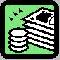Financial Terms Hedging

# Definition of Hedging## Hedging

A strategy designed to reduce investment risk using call options, put options, short selling, or futures
contracts. A hedge can help lock in existing profits. Its purpose is to reduce the volatility of a portfolio, by
reducing the risk of loss.

## Hedging

Reducing one's exposure to risk by buying and selling contracts for future delivery (of foreign currency, for example) at a price that is determined now.

# Related Terms:

## Cross hedging

The practice of hedging with a futures contract that is different from the underlying being
hedged.

## Dynamic hedging

A strategy that involves rebalancing hedge positions as market conditions change; a
strategy that seeks to insure the value of a portfolio using a synthetic put option.

## Hedging demands

Demands for securities to hedge particular sources of consumption risk, beyond the usual
mean-variance diversification motivation.

## Basis risk

The uncertainty about the basis at the time a hedge may be lifted. hedging substitutes basis risk for
price risk.

## Delta hedge

A dynamic hedging strategy using options with continuous adjustment of the number of options
used, as a function of the delta of the option.

## Forward sale

A method for hedging price risk which involves an agreement between a lender and an investor
to sell particular kinds of loans at a specified price and future time.## Hedge ratio (delta)

The ratio of volatility of the portfolio to be hedged and the return of the volatility of the
hedging instrument.

## Substitute sale

A method for hedging price risk that utilizes debt-market instruments, such as interest rate
futures, or that involves selling borrowed securities as the primary assets.

## Term structure

The relationship between the yields on fixed-interest
securities and their maturity dates. Expectation of changes in interest rates
affects term structure, as do liquidity preferences and hedging pressure. A
yield curve is one representation in the term structure.